Jul. 13, 2010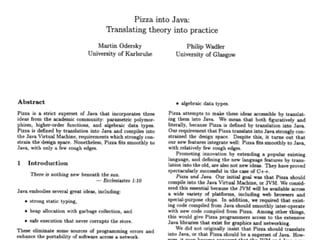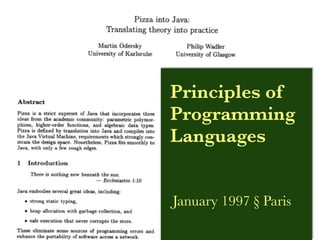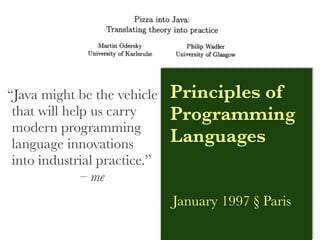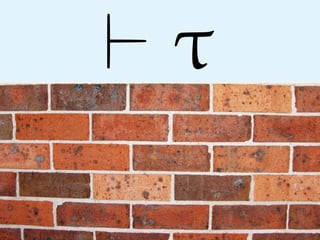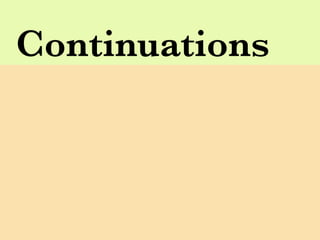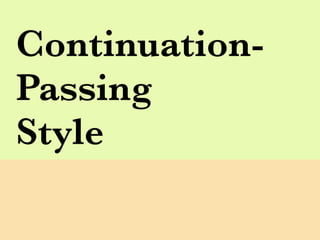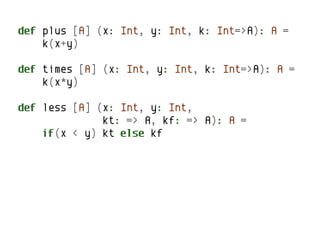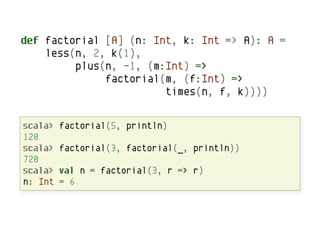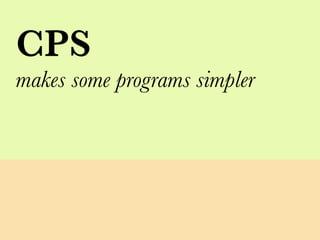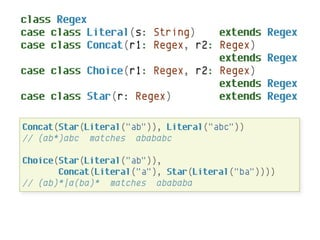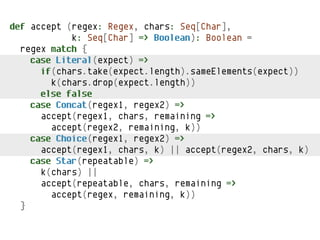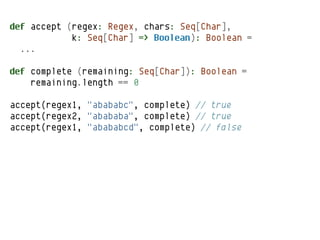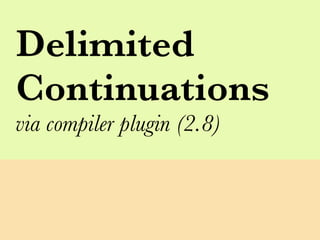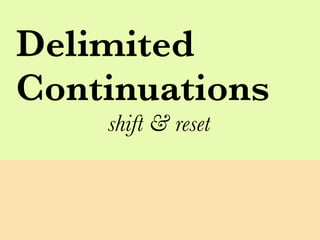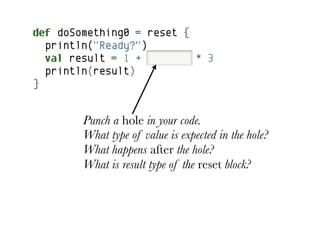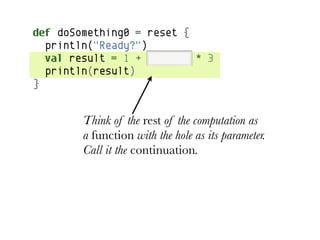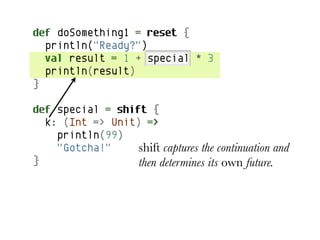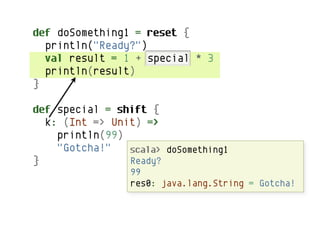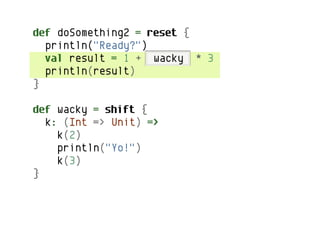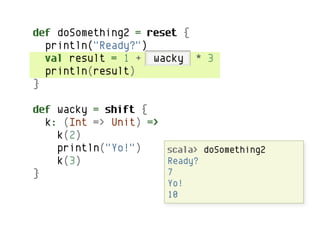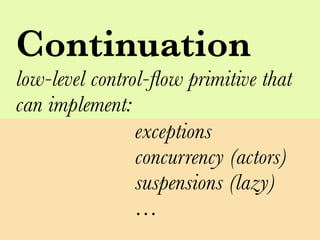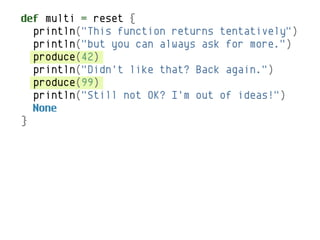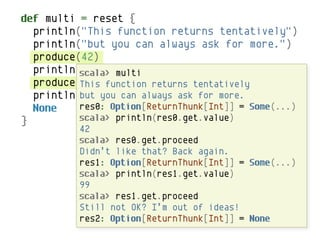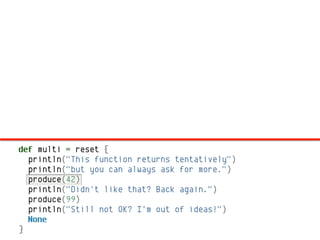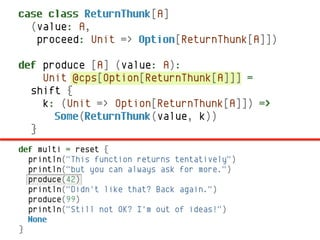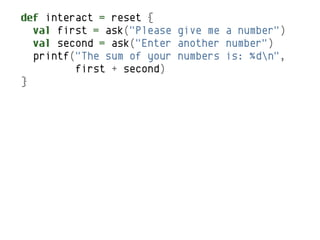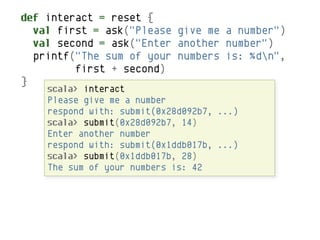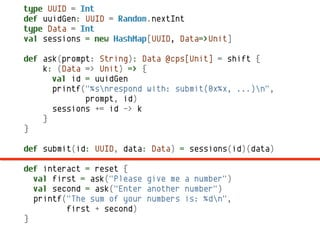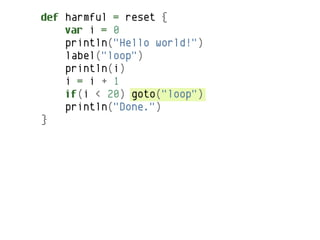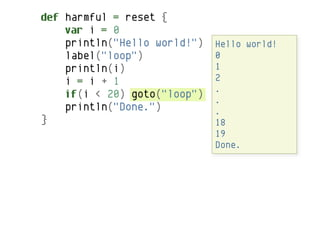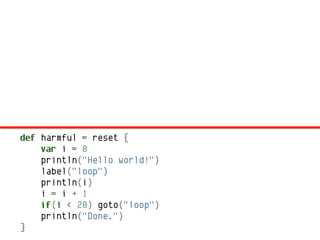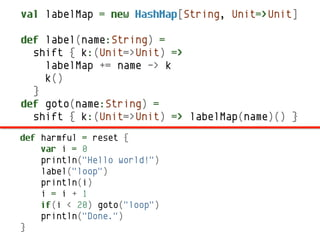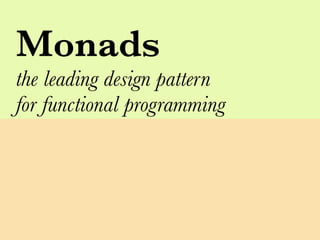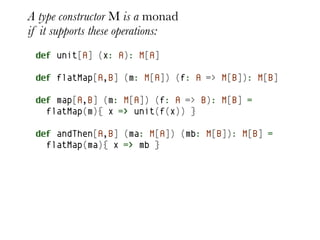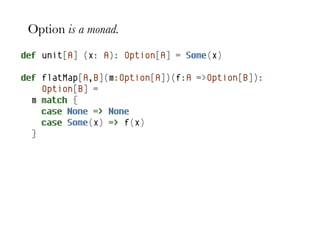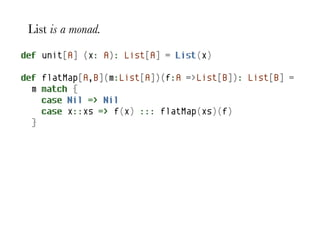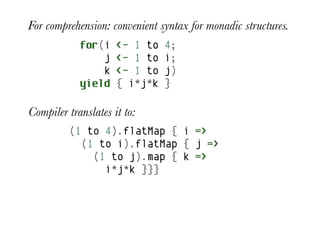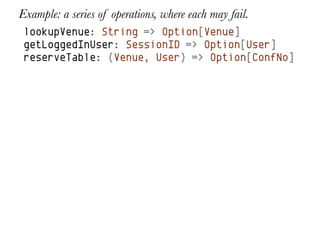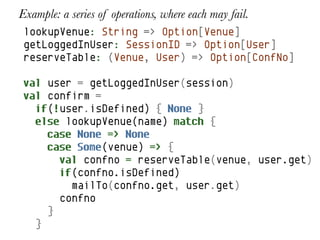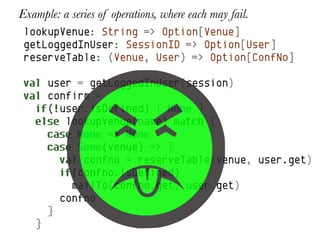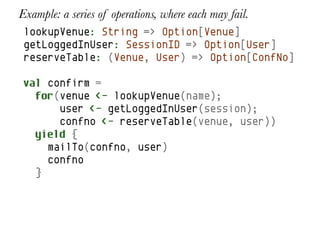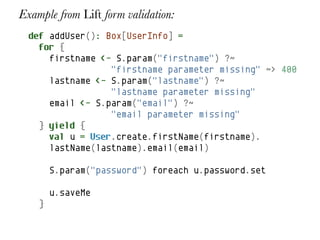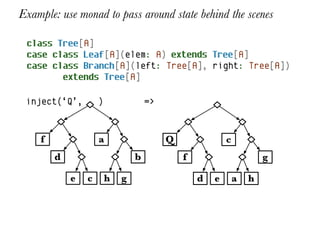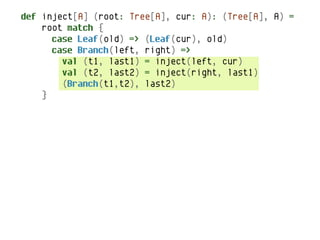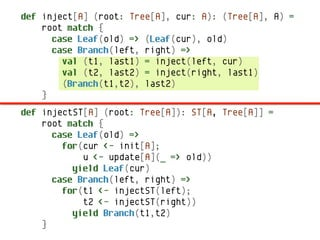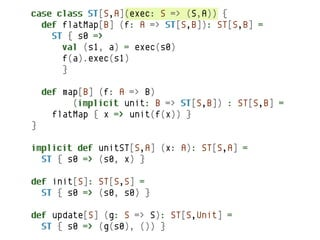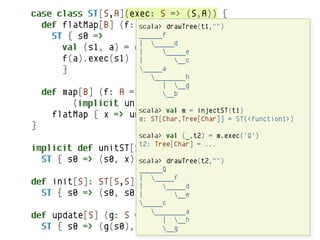1 of 58

• 1. Monadologie — professional help for type anxiety Christopher League 12 July 2010
• 2. Monadologie — github.com/league/scala-type-anxiety @chrisleague league@contrapunctus.net
• 7. “Java might be the vehicle Principles of that will help us carry Programming modern programming language innovations Languages into industrial practice.” – me January 1997 § Paris
• 15. def plus [A] (x: Int, y: Int, k: Int=>A): A = k(x+y) def times [A] (x: Int, y: Int, k: Int=>A): A = k(x*y) def less [A] (x: Int, y: Int, kt: => A, kf: => A): A = if(x < y) kt else kf
• 16. def factorial [A] (n: Int, k: Int => A): A = less(n, 2, k(1), plus(n, -1, (m:Int) => factorial(m, (f:Int) => times(n, f, k)))) scala> factorial(5, println) 120 scala> factorial(3, factorial(_, println)) 720 scala> val n = factorial(3, r => r) n: Int = 6
• 18. class Regex case class Literal(s: String) extends Regex case class Concat(r1: Regex, r2: Regex) extends Regex case class Choice(r1: Regex, r2: Regex) extends Regex case class Star(r: Regex) extends Regex Concat(Star(Literal("ab")), Literal("abc")) // (ab*)abc matches abababc Choice(Star(Literal("ab")), Concat(Literal("a"), Star(Literal("ba")))) // (ab)*|a(ba)* matches abababa
• 19. def accept (regex: Regex, chars: Seq[Char], k: Seq[Char] => Boolean): Boolean = regex match { case Literal(expect) => if(chars.take(expect.length).sameElements(expect)) k(chars.drop(expect.length)) else false case Concat(regex1, regex2) => accept(regex1, chars, remaining => accept(regex2, remaining, k)) case Choice(regex1, regex2) => accept(regex1, chars, k) || accept(regex2, chars, k) case Star(repeatable) => k(chars) || accept(repeatable, chars, remaining => accept(regex, remaining, k)) }
• 20. def accept (regex: Regex, chars: Seq[Char], k: Seq[Char] => Boolean): Boolean = ... def complete (remaining: Seq[Char]): Boolean = remaining.length == 0 accept(regex1, "abababc", complete) // true accept(regex2, "abababa", complete) // true accept(regex1, "abababcd", complete) // false
• 22. Delimited Continuations shift & reset
• 23. def doSomething0 = reset { println("Ready?") val result = 1 + special * 3 println(result) } Punch a hole in your code. What type of value is expected in the hole? What happens after the hole? What is result type of the reset block?
• 24. def doSomething0 = reset { println("Ready?") val result = 1 + special * 3 println(result) } Think of the rest of the computation as a function with the hole as its parameter. Call it the continuation.
• 25. def doSomething1 = reset { println("Ready?") val result = 1 + special * 3 println(result) } def special = shift { k: (Int => Unit) => println(99) "Gotcha!" shift captures the continuation and } then determines its own future.
• 26. def doSomething1 = reset { println("Ready?") val result = 1 + special * 3 println(result) } def special = shift { k: (Int => Unit) => println(99) "Gotcha!" shift doSomething1 scala> captures the continuation and } Ready?determines its own future. then 99 res0: java.lang.String = Gotcha!
• 27. def doSomething2 = reset { println("Ready?") val result = 1 + wacky * 3 println(result) } def wacky = shift { k: (Int => Unit) => k(2) println("Yo!") k(3) }
• 28. def doSomething2 = reset { println("Ready?") val result = 1 + wacky * 3 println(result) } def wacky = shift { k: (Int => Unit) => k(2) println("Yo!") scala> doSomething2 k(3) Ready? } 7 Yo! 10
• 29. Continuation low-level control-ﬂow primitive that can implement: exceptions concurrency (actors) suspensions (lazy) …
• 30. def multi = reset { println("This function returns tentatively") println("but you can always ask for more.") produce(42) println("Didn't like that? Back again.") produce(99) println("Still not OK? I'm out of ideas!") None }
• 31. def multi = reset { println("This function returns tentatively") println("but you can always ask for more.") produce(42) println("Didn't like that? Back again.") scala> multi produce(99) function returns tentatively This println("Still can alwaysI'm out more. but you not OK? ask for of ideas!") None res0: Option[ReturnThunk[Int]] = Some(...) } scala> println(res0.get.value) 42 scala> res0.get.proceed Didn’t like that? Back again. res1: Option[ReturnThunk[Int]] = Some(...) scala> println(res1.get.value) 99 scala> res1.get.proceed Still not OK? I’m out of ideas! res2: Option[ReturnThunk[Int]] = None
• 32. def multi = reset { println("This function returns tentatively") println("but you can always ask for more.") produce(42) println("Didn't like that? Back again.") produce(99) println("Still not OK? I'm out of ideas!") None }
• 33. case class ReturnThunk[A] (value: A, proceed: Unit => Option[ReturnThunk[A]]) def produce [A] (value: A): Unit @cps[Option[ReturnThunk[A]]] = shift { k: (Unit => Option[ReturnThunk[A]]) => Some(ReturnThunk(value, k)) } def multi = reset { println("This function returns tentatively") println("but you can always ask for more.") produce(42) println("Didn't like that? Back again.") produce(99) println("Still not OK? I'm out of ideas!") None }
• 34. def interact = reset { val first = ask("Please give me a number") val second = ask("Enter another number") printf("The sum of your numbers is: %dn", first + second) }
• 35. def interact = reset { val first = ask("Please give me a number") val second = ask("Enter another number") printf("The sum of your numbers is: %dn", first + second) } scala> interact Please give me a number respond with: submit(0x28d092b7, ...) scala> submit(0x28d092b7, 14) Enter another number respond with: submit(0x1ddb017b, ...) scala> submit(0x1ddb017b, 28) The sum of your numbers is: 42
• 36. type UUID = Int def uuidGen: UUID = Random.nextInt type Data = Int val sessions = new HashMap[UUID, Data=>Unit] def ask(prompt: String): Data @cps[Unit] = shift { k: (Data => Unit) => { val id = uuidGen printf("%snrespond with: submit(0x%x, ...)n", prompt, id) sessions += id -> k } } def submit(id: UUID, data: Data) = sessions(id)(data) def interact = reset { val first = ask("Please give me a number") val second = ask("Enter another number") printf("The sum of your numbers is: %dn", first + second) }
• 38. def harmful = reset { var i = 0 println("Hello world!") label("loop") println(i) i = i + 1 if(i < 20) goto("loop") println("Done.") }
• 39. def harmful = reset { var i = 0 println("Hello world!") Hello world! label("loop") 0 println(i) 1 i = i + 1 2 . if(i < 20) goto("loop") . println("Done.") . } 18 19 Done.
• 40. def harmful = reset { var i = 0 println("Hello world!") label("loop") println(i) i = i + 1 if(i < 20) goto("loop") println("Done.") }
• 41. val labelMap = new HashMap[String, Unit=>Unit] def label(name:String) = shift { k:(Unit=>Unit) => labelMap += name -> k k() } def goto(name:String) = shift { k:(Unit=>Unit) => labelMap(name)() } def harmful = reset { var i = 0 println("Hello world!") label("loop") println(i) i = i + 1 if(i < 20) goto("loop") println("Done.") }
• 43. A type constructor M is a monad if it supports these operations: def unit[A] (x: A): M[A] def flatMap[A,B] (m: M[A]) (f: A => M[B]): M[B] def map[A,B] (m: M[A]) (f: A => B): M[B] = flatMap(m){ x => unit(f(x)) } def andThen[A,B] (ma: M[A]) (mb: M[B]): M[B] = flatMap(ma){ x => mb }
• 44. Option is a monad. def unit[A] (x: A): Option[A] = Some(x) def flatMap[A,B](m:Option[A])(f:A =>Option[B]): Option[B] = m match { case None => None case Some(x) => f(x) }
• 45. List is a monad. def unit[A] (x: A): List[A] = List(x) def flatMap[A,B](m:List[A])(f:A =>List[B]): List[B] = m match { case Nil => Nil case x::xs => f(x) ::: flatMap(xs)(f) }
• 46. For comprehension: convenient syntax for monadic structures. for(i <- 1 to 4; j <- 1 to i; k <- 1 to j) yield { i*j*k } Compiler translates it to: (1 to 4).flatMap { i => (1 to i).flatMap { j => (1 to j).map { k => i*j*k }}}
• 47. Example: a series of operations, where each may fail. lookupVenue: String => Option[Venue] getLoggedInUser: SessionID => Option[User] reserveTable: (Venue, User) => Option[ConfNo]
• 48. Example: a series of operations, where each may fail. lookupVenue: String => Option[Venue] getLoggedInUser: SessionID => Option[User] reserveTable: (Venue, User) => Option[ConfNo] val user = getLoggedInUser(session) val confirm = if(!user.isDefined) { None } else lookupVenue(name) match { case None => None case Some(venue) => { val confno = reserveTable(venue, user.get) if(confno.isDefined) mailTo(confno.get, user.get) confno } }
• 49. Example: a series of operations, where each may fail. lookupVenue: String => Option[Venue] getLoggedInUser: SessionID => Option[User] reserveTable: (Venue, User) => Option[ConfNo] val user = getLoggedInUser(session) val confirm = if(!user.isDefined) { None } else lookupVenue(name) match { case None => None case Some(venue) => { val confno = reserveTable(venue, user.get) if(confno.isDefined) mailTo(confno.get, user.get) confno } }
• 50. Example: a series of operations, where each may fail. lookupVenue: String => Option[Venue] getLoggedInUser: SessionID => Option[User] reserveTable: (Venue, User) => Option[ConfNo] val confirm = for(venue <- lookupVenue(name); user <- getLoggedInUser(session); confno <- reserveTable(venue, user)) yield { mailTo(confno, user) confno }
• 51. Example from Lift form validation: def addUser(): Box[UserInfo] = for { firstname <- S.param("firstname") ?~ "firstname parameter missing" ~> 400 lastname <- S.param("lastname") ?~ "lastname parameter missing" email <- S.param("email") ?~ "email parameter missing" } yield { val u = User.create.firstName(firstname). lastName(lastname).email(email) S.param("password") foreach u.password.set u.saveMe }
• 52. Example: use monad to pass around state behind the scenes class Tree[A] case class Leaf[A](elem: A) extends Tree[A] case class Branch[A](left: Tree[A], right: Tree[A]) extends Tree[A] inject(‘Q’, ) => f a Q c d b f g e c h g d e a h
• 53. def inject[A] (root: Tree[A], cur: A): (Tree[A], A) = root match { case Leaf(old) => (Leaf(cur), old) case Branch(left, right) => val (t1, last1) = inject(left, cur) val (t2, last2) = inject(right, last1) (Branch(t1,t2), last2) }
• 54. def inject[A] (root: Tree[A], cur: A): (Tree[A], A) = root match { case Leaf(old) => (Leaf(cur), old) case Branch(left, right) => val (t1, last1) = inject(left, cur) val (t2, last2) = inject(right, last1) (Branch(t1,t2), last2) } def injectST[A] (root: Tree[A]): ST[A, Tree[A]] = root match { case Leaf(old) => for(cur <- init[A]; u <- update[A](_ => old)) yield Leaf(cur) case Branch(left, right) => for(t1 <- injectST(left); t2 <- injectST(right)) yield Branch(t1,t2) }
• 55. case class ST[S,A](exec: S => (S,A)) { def flatMap[B] (f: A => ST[S,B]): ST[S,B] = ST { s0 => val (s1, a) = exec(s0) f(a).exec(s1) } def map[B] (f: A => B) (implicit unit: B => ST[S,B]) : ST[S,B] = flatMap { x => unit(f(x)) } } implicit def unitST[S,A] (x: A): ST[S,A] = ST { s0 => (s0, x) } def init[S]: ST[S,S] = ST { s0 => (s0, s0) } def update[S] (g: S => S): ST[S,Unit] = ST { s0 => (g(s0), ()) }
• 56. case class ST[S,A](exec: S => (S,A)) { def flatMap[B] (f: scala> ST[S,B]): ST[S,B] = A => drawTree(t1,"") ST { s0 => ______f | _____d val (s1, a) = exec(s0) | _____e f(a).exec(s1) | __c } _____a ________h | __g def map[B] (f: A => B) __b (implicit unit: B => ST[S,B]) : ST[S,B] = flatMap { x => unit(f(x)) m }= injectST(t1) scala> val m: ST[Char,Tree[Char]] = ST(<function1>) } scala> val (_,t2) = m.exec('Q') t2: Tree[Char] = ... implicit def unitST[S,A] (x: A): ST[S,A] = ST { s0 => (s0, x) scala> drawTree(t2,"") } ______Q def init[S]: ST[S,S] | _____f = | _____d ST { s0 => (s0, s0)| } __e _____c ________a def update[S] (g: S => S): ST[S,Unit] | __h = ST { s0 => (g(s0), ()) } __g
• 58. Monadologie github.com/league/scala-type-anxiety @chrisleague league@contrapunctus.net